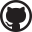# Poleiro

## Where the Coq lives

| Comments# Quotients in Coq

Quotients are crucial in mathematical practice, and it is a shame that they are not available in Coq's standard library. There was a recent discussion on the Coq GitHub page on this issue and the consequences of implementing quotients like Lean does, where the eliminator for function types has a reduction rule that breaks pleasant metatheoretic properties such as subject reduction.
In this post, we are going to define quotients in Coq with three standard axioms:
• Functional extensionality
• Propositional extensionality
• Constructive definite description (also known as the axiom of unique choice)

Check @functional_extensionality_dep :
∀ A B (f g : ∀ x : A, B x),
(∀ x : A, f x = g x) → f = g.

Check @propositional_extensionality :
∀ P Q, (PQ) → P = Q.

Check @constructive_definite_description :
∀ A P, (exists! x : A, P x) → {x : A | P x}.

As far as axioms go, these three are relatively harmless. In particular, they are valid in any elementary topos, which are generally regarded as a good universe for doing constructive, higher-order reasoning. (Naturally, adding axioms in type theory does not come for free: since they have no useful reduction behavior, our quotients won't compute.)

Section Quotient.

We define the quotient of T by an equivalence relation R as usual: it is the type of equivalence classes of R.

Context (T : Type) (R : relation T) (RP : Equivalence R).

Record quot := Quot_ {
quot_class : TProp;
quot_classP : ∃ x, quot_class = R x;
}.

The projection into the quotient is given by the Quot constructor below, which maps x to its equivalence class R x. This definition satisfies the usual properties: Quot x = Quot y if and only if R x y. The "if" direction requires the principle of proof irrelevance, which is a consequence of propositional extensionality.

Definition Quot (x : T) : quot :=
@Quot_ (R x) (ex_intro _ x erefl).

Lemma Quot_inj x y : Quot x = Quot yR x y.
Proof.
move=> e; rewrite -[R x y]/(quot_class (Quot x) y) e //=; reflexivity.
Qed.

Lemma eq_Quot x y : R x yQuot x = Quot y.
Proof.
move=> e; rewrite /Quot; move: (ex_intro _ y _).
suff ->: R y = R x.
move=> ?; congr Quot_; exact: proof_irrelevance.
apply: functional_extensionality=> z.
apply: propositional_extensionality.
by rewrite /= e.
Qed.

We can also show that Quot is surjective by extracting the witness in the existential.
Lemma Quot_inv q : ∃ x, q = Quot x.
Proof.
case: q=> [P [x xP]]; exists x; move: (ex_intro _ _ _).
rewrite xP=> e; congr Quot_; exact: proof_irrelevance.
Qed.

Unique choice comes into play when defining the elimination principles for the quotient. In its usual non-dependent form, the principle says that we can lift a function f : T S to another function quot S provided that f is constant on equivalence classes. We define a more general dependently typed version, which allows in particular to prove a property S q by proving that S (Quot x) holds for any x. The statement of the compatibility condition for f is a bit complicated because it needs to equate terms of different types S (Quot x) and S (Quot y), which requires us to transport the left-hand side along the equivalence R x y.

Section Elim.

Definition cast A B (e : A = B) : AB :=
match e with erefl => id end.

Context (S : quotType) (f : ∀ x, S (Quot x)).
Context (fP : ∀ x y (exy : R x y), cast (congr1 S (eq_Quot exy)) (f x) = f y).

We begin with an auxiliary result that uniquely characterizes the result of applying the eliminator to an element q : quot. Thanks to unique choice, this allows us to define the eliminator as a function quot_rect.

Lemma quot_rect_subproof (q : quot) :
exists! a : S q, ∃ x (exq : Quot x = q), a = cast (congr1 S exq) (f x).
Proof.
case: (Quot_inv q)=> x -> {q}.
exists (f x); split=> [|a]; first by exists x, erefl.
case=> y [eyx -> {a}].
by rewrite (proof_irrelevance _ eyx (eq_Quot (Quot_inj eyx))) fP.
Qed.

Definition quot_rect q : S q :=
sval (constructive_definite_description _ (quot_rect_subproof q)).

Lemma quot_rectE x : quot_rect (Quot x) = f x.
Proof.
rewrite /quot_rect.
case: constructive_definite_description=> _ [y [eyx /= ->]].
by rewrite (proof_irrelevance _ eyx (eq_Quot (Quot_inj eyx))) fP.
Qed.

End Elim.

In the non-dependent case, the compatibility condition acquires its usual form.

Section Rec.

Context S (f : TS) (fP : ∀ x y, R x yf x = f y).

Definition congr1CE (A B : Type) (b : B) x y (e : x = y) :
congr1 (λ _ : A, b) e = erefl :=
match e with erefl => erefl end.

Definition quot_rec : quot -> S :=
@quot_rect (λ _, S) f
(λ x y exy, etrans
(congr1 (λ p, cast p (f x)) (congr1CE S (eq_Quot exy)))
(fP exy)).

Lemma quot_recE x : quot_rec (Quot x) = f x.
Proof. by rewrite /quot_rec quot_rectE. Qed.

End Rec.

End Quotient.
comments powered by Disqus